Maths Index

Properties of plane Shapes

Cx,Cy ...Centroid

The middle point of a geometric figure.   The coordinates of a point in a figure, which are at the average distance from the coordinates of all points on the surface of the figure.   If the figure is 2-dimensional, the applicable term is, centre of area, if 3-dimensional the terms are, centre of volume, or centre of mass.   If figure is considered to be a particle then all of the mass or area is assumed to act at this point.

I... Moment of Inertia. /Second Moment of Area

When assessing the strength of beams to bending the "Area Moment Of Inertia" of a beams cross section indicates the beams ability to resist bending.   The larger the Second Moment of Area the less the beam will bend.

The Second Moment of Area is a geometrical property of a beam and depends on a reference axis ( which is in the plane of the area).   The smallest Second Moment of Area about any axis passes through the centroid.   If the area is composed of an infinite number of small areas da the Second Moment of Area around an axis is the sum of all these areas x (the distance of the area da from the axis) 2...This is a distance from a line

J.. Polar Moment of Inertia of a plane area

The polar moment of inertia relates to an axis which is perpendicular to the plane of an area.

If all of the area is assumed to comprise of infinitely small areas da then the polar moment of inertia is the sum of all of these areas x .r2
r = the radius of da from the perpendicular axis - for a plane area the perpendicular axis is a point

The polar moment of inertia is the sum of any two moments of inertia about axes at right angles to each other e.g.

J = Ixx + Iyy

When considering solids the Polar Moment of inertia is a measure of the resistance of a mass to angular acceleration.

Parallel Axis Theory
To determine the Second Moment of Area about an axis which is parallel to a centroid axis and at a distance k .

Iw = I + A.k2

Notes

Cx , Cy = Centroid Co-ordinates.
I xx, I yy are the Moments of Area for axes through the centroid C in the direction x,y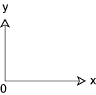Area = A Cx Cy I xx I yy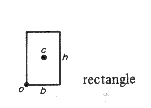b.h b /2 h /2 A.h 2 / 12 A.b 2 / 12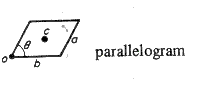a.b.sinθ ( b + a.cosθ ) / 2a.sinθ / 2 A.(a sin θ ) 2 / 12 A.(b 2 + a 2 cos 2 θ ) / 12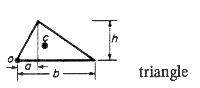b.h/2 (a +b)/3 h/3 A.h 2/18 A. (b 2 - a.b + a. 2 ) / 18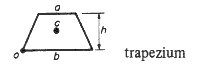h.(a + b)/2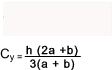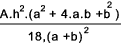-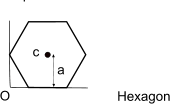6.a2 .tan(30o) = 3,464 a2 a /cos(30o) = 1.155 a a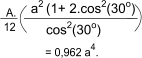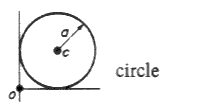π.a2 aa A.a 2 /4 A.a 2 /4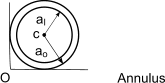π.(ao2 - ai2 ) a oa o π.(ao4 - ai4 )/4 π.(ao4 - ai4 )/4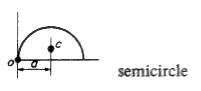π.a2 /2 a 4.a /3.π A.a 2 (9π 2 - 64 ) / 36.π 2           = 0,1098a 4 A.a 2 /8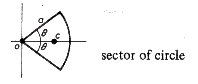a 2.θ 2.a.sin θ / 3.θ 0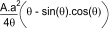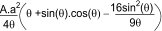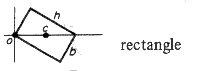b.h Sqrt(b2 + h2 ) /2 0/ A.b 2.h 2 / 6.( h 2 + b 2 ) A.(h 4+ b 4 ) / 12.( h 2+ b 2)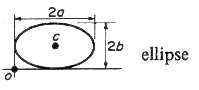π.a.b a b A.b2 / 4 A.a 2 /4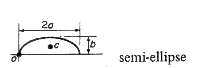π.a.b /2 a 4b / 3π A.b 2 (9.π 2 - 64 ) / 36 π 2 A.a 2 /4

 Links to Properties of Figures Wolfram - M.of I. of common solids..High quality information Efunda - Common solids..Very clear comprehensive information on all important properties of solid shapes Chapter 5= Moment on Inertia..Download of useful review notes

Maths Index